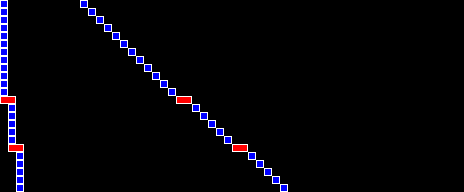# BRR Lines

## A new line drawing method for the cycle savvy

In the demoscene, there's never a boundary that can't be pushed. My recent 4k demo "Boo!" (YouTube), released at X 2023, is a humble attempt to contribute to this ongoing evolution. In particular, it pioneers a new way of computing lines.

Apart from unlocking a number of improvements in XOR fillers and similar effects, the method, as used in the 4k, also significantly raises the bar for 50hz sprite-filled 3D cubes (SMGF). The cube displayed in "Boo!" draws over an area of size 172x172, up from 140x140 in Protogeo 100%. This improvement to size is because line computation that previously either took 7+ cycles per pixel (or exhaustive amounts of memory) can now, thanks to the BRR ("bit reverse rendered") line method, done in just 5 cycles per pixel.

Here, a line is a linear interpolation from a value x1 to a value x2 over y steps. There has been, through the history of the demoscene, an exuberant number of approaches to solving this problem. The default, typically used in XOR fillers, adds a 16 bit slope to a running position at every step. A similar approach, "Bresenham", only works for slopes with an integer part of 0, but does away with the need for division by multiplying all formulas with the denominator, and thus changing the necessary overflow check from the "power of 2" boundary (256) to one against dy. Other methods include fractional precalculation which calculates the bit pattern of when the fractional part of the position overflows, or full precalculation, which stores all potential slopes in memory. The latter leverages the fact that steeper slopes can only appear for shorter lines, thereby only storing the necessary length.

All these methods are slower than BRR lines, for the step-by-step computation of the positions:

16 bit slope Bresenham Fractional precomputation Full slope precalculation BRR Lines
```tya
tay
txa
tax
```
```adc zp
bcc
sbc zp
inx
```
```lsr zp
```
```adc (zp), y
iny
```
```cpx #imm
```
14 cycles 8-10 cycles 8 cycles 7 cycles 5 cycles

Note that as far as the slope math goes, Bresenham is special since it doesn't require the division of x by y, but BRR lines do. So for short line segments, Bresenham can still "win". In fact, BRR lines also need a bit more elaborate math for the remainder. (So this method really shines if you're able to pull in the 1541 for help. See my upcoming post about drivecode)

How does this work? Let's dive deeper. First off, the idea (used in fractional precomputation) of treating the computation of integer part and fractional part of the position as different kinds of computations is a good, since they do tend to behave differently:Here, to the very left is a line with only an integer slope (0 and 1), and then to the right with progressively larger fractional slope components. Note how the integer slope yields a regular pattern, while the fractional slope introduces additional, irregular, notches.

We've been assuming that we want to space said notches evenly. Do we have to? Turns out we don't! Here are the same lines, with one notch at 1/2, and the other at 1/4:These still look like lines.

This is exactly the method used by BRR lines. Instead of putting the notch at positions that depend on the slope (fractional part of x/y), we instead only take the remainder of x divided by y, which tells us how many notches we need. And now we use a comparison operation that gives us exactly that many notches over a distance of y.So every time a value generated from the slope is larger than the value at the pattern, we introduce a notch. The pattern can in theory be any list of (non-repeating) numbers (even a random permutation of 0..255 "works"), but I've found that the one pattern that mimicks real line slopes the best is the bitreverse, which is what is used above.

And this is how BRR lines (right) look compared to traditional line drawing (left):You will have noticed that the slope-based lines above "wiggle" more. This is true, and might be considered one of the drawbacks of the algorithm. However, the fact that BRR lines are more temporally stable can also conceivably be useful in some applications.

So if the cpx compares against the bitreverse, what's "x"? It's a value derived from the remainder of x/y. In particular, it's (assuming you're drawing from y1 to y2, and interpolating from x1 to x2):

``````sorted(bitreverse[y1:y2])[(x2-x1) % (y2 - y1)]
``````

This looks very expensive, but it's not. You do have to divide to get the modulo, but you're doing that anyway since you need the integer part of the slope. And you don't actually need to sort - since you're only looking for a single value in the sorted list, you can instead do a bit-wise quickselect. "Boo!" computes up to nine line slopes, including the above formula, per frame. (In the drive)

I've so far implemented two different bit-fiddling approaches for computing the above, the Python version of which you can download here. (Or you can dig into the drive code of "Boo" to find the 6502 version of the algorithm. The bitreverse comparison value is computed at \$0401, and is based on the second implementation.)

So there you are. Lines are cheap now! "Boo!" demonstrates how that changes the playing field in sprite vectors, but they can be used in quite a few other effects. (Hint: what else interpolates from a to b?)